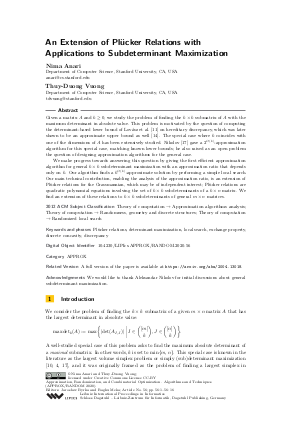Document# An Extension of Plücker Relations with Applications to Subdeterminant Maximization

### Authors Nima Anari, Thuy-Duong Vuong## File

LIPIcs.APPROX-RANDOM.2020.56.pdf
• Filesize: 494 kB
• 16 pages

## Acknowledgements

We would like to thank Aleksandar Nikolov for initial discussions about general subdeterminant maximization.

## Cite As

Nima Anari and Thuy-Duong Vuong. An Extension of Plücker Relations with Applications to Subdeterminant Maximization. In Approximation, Randomization, and Combinatorial Optimization. Algorithms and Techniques (APPROX/RANDOM 2020). Leibniz International Proceedings in Informatics (LIPIcs), Volume 176, pp. 56:1-56:16, Schloss Dagstuhl - Leibniz-Zentrum für Informatik (2020)
https://doi.org/10.4230/LIPIcs.APPROX/RANDOM.2020.56

## Abstract

Given a matrix A and k ≥ 0, we study the problem of finding the k × k submatrix of A with the maximum determinant in absolute value. This problem is motivated by the question of computing the determinant-based lower bound of cite{LSV86} on hereditary discrepancy, which was later shown to be an approximate upper bound as well [Matoušek, 2013]. The special case where k coincides with one of the dimensions of A has been extensively studied. Nikolov gave a 2^{O(k)}-approximation algorithm for this special case, matching known lower bounds; he also raised as an open problem the question of designing approximation algorithms for the general case. We make progress towards answering this question by giving the first efficient approximation algorithm for general k× k subdeterminant maximization with an approximation ratio that depends only on k. Our algorithm finds a k^{O(k)}-approximate solution by performing a simple local search. Our main technical contribution, enabling the analysis of the approximation ratio, is an extension of Plücker relations for the Grassmannian, which may be of independent interest; Plücker relations are quadratic polynomial equations involving the set of k× k subdeterminants of a k× n matrix. We find an extension of these relations to k× k subdeterminants of general m× n matrices.

## Subject Classification

##### ACM Subject Classification
• Theory of computation → Approximation algorithms analysis
• Theory of computation → Randomness, geometry and discrete structures
• Theory of computation → Randomized local search
##### Keywords
• Plücker relations
• determinant maximization
• local search
• exchange property
• discrete concavity
• discrepancy

## Metrics

• Access Statistics
• Total Accesses (updated on a weekly basis)
0

## References

1. Nima Anari, Kuikui Liu, Shayan Oveis Gharan, and Cynthia Vinzant. Log-concave polynomials iv: Exchange properties, tight mixing times, and faster sampling of spanning trees. arXiv preprint, 2020. URL: http://arxiv.org/abs/2004.07220.
2. Nima Anari and Shayan Oveis Gharan. A generalization of permanent inequalities and applications in counting and optimization. In Proceedings of the 49th Annual ACM SIGACT Symposium on Theory of Computing, pages 384-396. ACM, June 2017. URL: https://doi.org/10.1145/3055399.3055469.
3. Nima Anari, Shayan Oveis Gharan, and Cynthia Vinzant. Log-concave polynomials i: Entropy and a deterministic approximation algorithm for counting bases of matroids. In Proceedings of the 59th IEEE Annual Symposium on Foundations of Computer Science. IEEE Computer Society, October 2018. URL: https://doi.org/10.1109/focs.2018.00013.
4. Marco Di Summa, Friedrich Eisenbrand, Yuri Faenza, and Carsten Moldenhauer. On largest volume simplices and sub-determinants. In Proceedings of the twenty-sixth annual ACM-SIAM symposium on Discrete algorithms, pages 315-323. SIAM, 2014.5. Andreas Dress and Walter Wenzel. Grassmann-plücker relations and matroids with coefficients. Advances in Mathematics, 86(1):68-110, 1991.6. Javad B Ebrahimi, Damian Straszak, and Nisheeth K Vishnoi. Subdeterminant maximization via nonconvex relaxations and anti-concentration. In 2017 IEEE 58th Annual Symposium on Foundations of Computer Science (FOCS), pages 1020-1031. Ieee, 2017.7. Valerii Vadimovich Fedorov. Theory of optimal experiments. Elsevier, 2013.8. Piotr Indyk, Sepideh Mahabadi, Shayan Oveis Gharan, and Alireza Rezaei. Composable core-sets for determinant maximization problems via spectral spanners. In Proceedings of the Fourteenth Annual ACM-SIAM Symposium on Discrete Algorithms, pages 1675-1694. SIAM, 2020.9. Tarun Kathuria and Amit Deshpande. On sampling and greedy map inference of constrained determinantal point processes. arXiv preprint, 2016. URL: http://arxiv.org/abs/1607.01551.
10. Leonid Khachiyan. On the complexity of approximating extremal determinants in matrices. Journal of Complexity, 11(1):138-153, 1995.11. László Lovász, Joel Spencer, and Katalin Vesztergombi. Discrepancy of set-systems and matrices. European Journal of Combinatorics, 7(2):151-160, 1986.12. Vivek Madan, Aleksandar Nikolov, Mohit Singh, and Uthaipon Tantipongpipat. Maximizing determinants under matroid constraints. arXiv preprint, 2020. URL: http://arxiv.org/abs/2004.07886.
13. Vivek Madan, Mohit Singh, Uthaipon Tantipongpipat, and Weijun Xie. Combinatorial algorithms for optimal design. In Conference on Learning Theory, pages 2210-2258, 2019.14. Jiří Matoušek. The determinant bound for discrepancy is almost tight. Proceedings of the American Mathematical Society, 141(2):451-460, 2013.15. Jiří Matoušek, Aleksandar Nikolov, and Kunal Talwar. Factorization norms and hereditary discrepancy. International Mathematics Research Notices, 2020(3):751-780, 2020.16. Kazuo Murota. Discrete convex analysis. SIAM, 2003.17. Aleksandar Nikolov. Randomized rounding for the largest simplex problem. In Proceedings of the forty-seventh annual ACM symposium on Theory of computing, pages 861-870, 2015.18. Aleksandar Nikolov and Mohit Singh. Maximizing determinants under partition constraints. In Proceedings of the forty-eighth annual ACM symposium on Theory of Computing, pages 192-201, 2016.19. Aleksandar Nikolov and Kunal Talwar. Approximating hereditary discrepancy via small width ellipsoids. In Proceedings of the twenty-sixth annual ACM-SIAM symposium on Discrete algorithms, pages 324-336. SIAM, 2014.20. Asa Packer. Polynomial-time approximation of largest simplices in v-polytopes. Discrete Applied Mathematics, 134:213-237, January 2004. URL: https://doi.org/10.1016/S0166-218X(03)00226-9.
21. Dömötör Pálvölgyi. Indecomposable coverings with concave polygons. Discrete & Computational Geometry, 44(3):577-588, 2010.22. Damian Straszak and Nisheeth K Vishnoi. Real stable polynomials and matroids: Optimization and counting. In Proceedings of the 49th Annual ACM SIGACT Symposium on Theory of Computing, pages 370-383, 2017.X

Feedback for Dagstuhl Publishing